# Study of solutions to an initial and boundary value problem for certain systems with variable exponents

## Abstract

In this paper, the existence and blow-up property of solutions to an initial and boundary value problem for a nonlinear parabolic system with variable exponents is studied. Meanwhile, the blow-up property of solutions for a nonlinear hyperbolic system is also obtained.

## 1 Introduction

In this paper, we first consider the initial and boundary value problem to the following nonlinear parabolic system with variable exponents:

$\left\{\begin{array}{c}{u}_{t}=\mathrm{\Delta }u+{f}_{1}\left(u,v\right),\phantom{\rule{1em}{0ex}}\left(x,t\right)\in {Q}_{T},\hfill \\ {v}_{t}=\mathrm{\Delta }v+{f}_{2}\left(u,v\right),\phantom{\rule{1em}{0ex}}\left(x,t\right)\in {Q}_{T},\hfill \\ u\left(x,t\right)=0,\phantom{\rule{2em}{0ex}}v\left(x,t\right)=0,\phantom{\rule{1em}{0ex}}\left(x,t\right)\in {S}_{T},\hfill \\ u\left(x,0\right)={u}_{0}\left(x\right),\phantom{\rule{2em}{0ex}}v\left(x,0\right)={v}_{0}\left(x\right),\phantom{\rule{1em}{0ex}}x\in \mathrm{\Omega },\hfill \end{array}$
(1.1)

where $\mathrm{\Omega }\subset {\mathbb{R}}^{N}$ is a bounded domain with smooth boundary Ω and $0, ${Q}_{T}=\mathrm{\Omega }×\left[0,T\right)$, ${S}_{T}$ denotes the lateral boundary of the cylinder ${Q}_{T}$, and the source terms ${f}_{1}$, ${f}_{2}$ are in the form

${f}_{1}\left(u,v\right)={a}_{1}\left(x\right){v}^{{p}_{1}\left(x\right)}\phantom{\rule{1em}{0ex}}\text{and}\phantom{\rule{1em}{0ex}}{f}_{2}\left(u,v\right)={a}_{2}\left(x\right){u}^{{p}_{2}\left(x\right)},$

or

${f}_{1}\left(u,v\right)={a}_{1}\left(x\right){\int }_{\mathrm{\Omega }}{v}^{{p}_{1}\left(y\right)}\left(y,t\right)\phantom{\rule{0.2em}{0ex}}dy\phantom{\rule{1em}{0ex}}\text{and}\phantom{\rule{1em}{0ex}}{f}_{2}\left(u,v\right)={a}_{2}\left(x\right){\int }_{\mathrm{\Omega }}{u}^{{p}_{2}\left(y\right)}\left(y,t\right)\phantom{\rule{0.2em}{0ex}}dy,$

respectively, where ${p}_{1}$, ${p}_{2}$, ${a}_{1}$, ${a}_{2}$ are functions satisfying conditions (2.1) below.

In the case when ${p}_{1}$, ${p}_{2}$ are constants, system (1.1) provides a simple example of a reaction-diffusion system. It can be used as a model to describe heat propagation in a two-component combustible mixture. There have been many results about the existence, boundedness and blow-up properties of the solutions; we refer the readers to the bibliography given in .

The motivation of this work is due to , where the following system of equations is studied.

$\left\{\begin{array}{c}{u}_{t}-\mathrm{\Delta }u={v}^{p},\hfill \\ {v}_{t}-\mathrm{\Delta }v={u}^{q},\hfill \end{array}$
(1.2)

where $x\in {\mathbb{R}}^{N}$ ($N\ge 1$), $t>0$, and p, q are positive numbers. The authors investigated the boundedness and blow-up of solutions to problem (1.2). Furthermore, the authors also studied the uniqueness and global existence of solutions (see ).

Besides, this work is also motivated by  in which the following problem is considered:

$\left\{\begin{array}{c}{u}_{t}=\mathrm{\Delta }u+f\left(x,u\right),\phantom{\rule{1em}{0ex}}\left(x,t\right)\in \mathrm{\Omega }×\left[0,T\right),\hfill \\ u\left(x,0\right)={u}_{0}\left(x\right),\phantom{\rule{1em}{0ex}}x\in \mathrm{\Omega },\hfill \\ u\left(x,t\right)=0,\phantom{\rule{1em}{0ex}}\left(x,t\right)\in \partial \mathrm{\Omega }×\left[0,T\right),\hfill \end{array}$
(1.3)

where $\mathrm{\Omega }\in {\mathbb{R}}^{n}$ is a bounded domain with smooth boundary Ω, and the source term is of the form $f\left(x,u\right)=a\left(x\right){u}^{p\left(x\right)}$ or $f\left(x,u\right)=a\left(x\right){\int }_{\mathrm{\Omega }}{u}^{q\left(y\right)}\left(y,t\right)\phantom{\rule{0.2em}{0ex}}dy$. The author studied the blow-up property of solutions for parabolic and hyperbolic problems. Parabolic problems with sources like the ones in (1.3) appear in several branches of applied mathematics, which can be used to model chemical reactions, heat transfer or population dynamics etc. We also refer the interested reader to  and the references therein.

We also study the following nonlinear hyperbolic system of equations:

$\left\{\begin{array}{c}{u}_{tt}=\mathrm{\Delta }u+{f}_{1}\left(u,v\right),\phantom{\rule{1em}{0ex}}\left(x,t\right)\in {Q}_{T},\hfill \\ {v}_{tt}=\mathrm{\Delta }v+{f}_{2}\left(u,v\right),\phantom{\rule{1em}{0ex}}\left(x,t\right)\in {Q}_{T},\hfill \\ u\left(x,t\right)=0,\phantom{\rule{2em}{0ex}}v\left(x,t\right)=0,\phantom{\rule{1em}{0ex}}\left(x,t\right)\in {S}_{T},\hfill \\ u\left(x,0\right)={u}_{0}\left(x\right),\phantom{\rule{2em}{0ex}}v\left(x,0\right)={v}_{0}\left(x\right),\phantom{\rule{1em}{0ex}}x\in \mathrm{\Omega },\hfill \\ {u}_{t}\left(x,0\right)={u}_{1}\left(x\right),\phantom{\rule{2em}{0ex}}{v}_{t}\left(x,0\right)={v}_{1}\left(x\right),\phantom{\rule{1em}{0ex}}x\in \mathrm{\Omega }.\hfill \end{array}$
(1.4)

The aim of this paper is to extend the results in [2, 8] to the case of parabolic system (1.1) and hyperbolic system (1.4). As far as we know, this seems to be the first paper, where the blow-up phenomenon is studied with variable exponents for the initial and boundary value problem to some parabolic and hyperbolic systems. The main method of the proof is similar to that in [3, 8].

We conclude this introduction by describing the outline of this paper. Some preliminary results, including existence of solutions to problem (1.1), are gathered in Section 2. The blow-up property of solutions are stated and proved in Section 3. Finally, in Section 4, we prove the blow-up property of solutions for hyperbolic problem (1.4).

## 2 Existence of solutions

In this section, we first state some assumptions and definitions needed in the proof of our main result and then prove the existence of solutions.

Throughout the paper, we assume that the exponents ${p}_{1}\left(x\right),{p}_{2}\left(x\right):\mathrm{\Omega }\to \left(1,+\mathrm{\infty }\right)$ and the continuous functions ${a}_{1}\left(x\right),{a}_{2}\left(x\right):\mathrm{\Omega }\to \mathbb{R}$ satisfy the following conditions: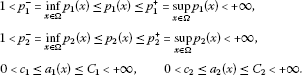(2.1)

Definition 2.1 We say that the solution $\left(u\left(x,t\right),v\left(x,t\right)\right)$ for problem (1.1) blows up in finite time if there exists an instant ${T}^{\ast }<\mathrm{\infty }$ such that

where

$⦀\left(u,v\right)⦀=\underset{t\in \left[0,T\right)}{sup}\left\{{\parallel u\left(\cdot ,t\right)\parallel }_{\mathrm{\infty }}+{\parallel v\left(\cdot ,t\right)\parallel }_{\mathrm{\infty }}\right\}.$

Our first result here is the following.

Theorem 2.1 Let $\mathrm{\Omega }\subset {\mathbb{R}}^{N}$ be a bounded smooth domain, ${p}_{1}\left(x\right)$, ${p}_{2}\left(x\right)$, ${a}_{1}\left(x\right)$, ${a}_{2}\left(x\right)$ satisfy the conditions in (2.1), and assume that ${u}_{0}\left(x\right)$ and ${v}_{0}\left(x\right)$ are nonnegative, continuous and bounded. Then there exists a ${T}^{0}$, $0<{T}^{0}\le \mathrm{\infty }$, such that problem (1.1) has a nonnegative and bounded solution $\left(u,v\right)$ in ${Q}_{{T}^{0}}$.

Proof We only prove the case when ${f}_{1}\left(u,v\right)={a}_{1}\left(x\right){v}^{{p}_{1}\left(x\right)}$ and ${f}_{2}\left(u,v\right)={a}_{2}\left(x\right){u}^{{p}_{2}\left(x\right)}$, and the proofs to the cases ${f}_{1}\left(u,v\right)={a}_{1}\left(x\right){\int }_{\mathrm{\Omega }}{v}^{{p}_{1}\left(y\right)}\left(y,t\right)\phantom{\rule{0.2em}{0ex}}dy$ and ${f}_{2}\left(u,v\right)={a}_{2}\left(x\right){\int }_{\mathrm{\Omega }}{u}^{{p}_{2}\left(y\right)}\left(y,t\right)\phantom{\rule{0.2em}{0ex}}dy$ are similar.

Let us consider the equivalent systems of (1.1)

$\left\{\begin{array}{c}u\left(x,t\right)={\int }_{\mathrm{\Omega }}g\left(x,y,t\right){u}_{0}\left(y\right)\phantom{\rule{0.2em}{0ex}}dy+{\int }_{0}^{t}{\int }_{\mathrm{\Omega }}g\left(x,y,t-s\right){a}_{1}\left(y\right){v}^{{p}_{1}\left(y\right)}\phantom{\rule{0.2em}{0ex}}dy\phantom{\rule{0.2em}{0ex}}ds,\hfill \\ v\left(x,t\right)={\int }_{\mathrm{\Omega }}g\left(x,y,t\right){v}_{0}\left(y\right)\phantom{\rule{0.2em}{0ex}}dy+{\int }_{0}^{t}{\int }_{\mathrm{\Omega }}g\left(x,y,t-s\right){a}_{2}\left(y\right){u}^{{p}_{2}\left(y\right)}\phantom{\rule{0.2em}{0ex}}dy\phantom{\rule{0.2em}{0ex}}ds,\hfill \end{array}$

where $g\left(x,y,t\right)$ is the corresponding Green function. Then the existence and uniqueness of solutions for a given $\left({u}_{0}\left(x\right),{v}_{0}\left(x\right)\right)$ could be obtained by a fixed point argument.

We introduce the following iteration scheme: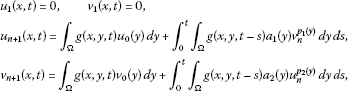and the convergence of the sequence $\left\{\left({u}_{n},{v}_{n}\right)\right\}$ follows by showing that

$\left\{\begin{array}{c}{\mathrm{\Phi }}_{1}\left(v\right)={\int }_{0}^{t}{\int }_{\mathrm{\Omega }}g\left(x,y,t-s\right){a}_{1}\left(y\right){v}_{n}^{{p}_{1}\left(y\right)}\phantom{\rule{0.2em}{0ex}}dy\phantom{\rule{0.2em}{0ex}}ds,\hfill \\ {\mathrm{\Phi }}_{2}\left(u\right)={\int }_{0}^{t}{\int }_{\mathrm{\Omega }}g\left(x,y,t-s\right){a}_{2}\left(y\right){u}_{n}^{{p}_{2}\left(y\right)}\phantom{\rule{0.2em}{0ex}}dy\phantom{\rule{0.2em}{0ex}}ds\hfill \end{array}$

is a contraction in the set ${E}_{T}$ to be defined below.

Now, we define

$\mathrm{\Psi }\left(u,v\right)=\left({\mathrm{\Phi }}_{1}\left(v\right),{\mathrm{\Phi }}_{2}\left(u\right)\right),$

whereWe denote

$\mathrm{\Psi }\left(u,v\right)-\mathrm{\Psi }\left(w,z\right)=\left({\mathrm{\Phi }}_{1}\left(v\right)-{\mathrm{\Phi }}_{1}\left(z\right),{\mathrm{\Phi }}_{2}\left(u\right)-{\mathrm{\Phi }}_{2}\left(w\right)\right),$

and for arbitrary $T>0$, define the set

${E}_{T}=\left\{{C}^{1,2}\left({\mathrm{\Omega }}_{T}\right)\cap C\left(\overline{{\mathrm{\Omega }}_{T}}\right)|⦀\left(u,v\right)⦀\le M\right\},$

where ${\mathrm{\Omega }}_{T}=\mathrm{\Omega }×\left[0,T\right]$, $M>⦀\left({u}_{0}\left(x\right),{v}_{0}\left(x\right)\right)⦀$ is a fixed positive constant.

We claim that Ψ is a contraction on ${E}_{T}$. In fact, for any $x\in \mathrm{\Omega }$ fixed, we have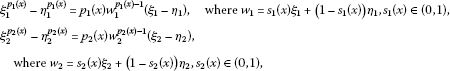and we always have

$⦀{p}_{i}\left(x\right){w}_{i}^{{p}_{i}\left(x\right)-1}\left({\xi }_{i}-{\eta }_{i}\right)⦀\le {p}_{i}^{+}{\left(2M\right)}^{{p}_{i}^{+}-1}{\parallel {\xi }_{i}-{\eta }_{i}\parallel }_{\mathrm{\infty }},\phantom{\rule{1em}{0ex}}i=1,2.$
(2.2)

Now, we define

$\mu \left(t\right)=\underset{x\in \overline{\mathrm{\Omega }},0\le \tau

It is obvious that $\mu \left(t\right)\to 0$ when $t\to {0}^{+}$.

Then, by using inequality (2.2), we get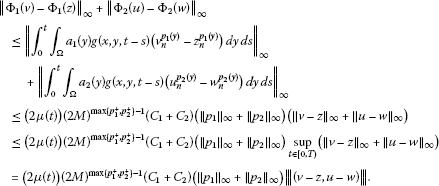Hence, for sufficiently small t, we have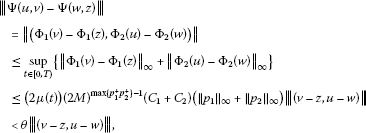where $\theta <1$ is a constant. Then Ψ is a strict contraction. □

## 3 Blow-up of solutions

In this section, we study the blow-up property of the solutions to problem (1.1). We need the following lemma.

Lemma 3.1 Let $y\left(t\right)$ be a solution of

${y}^{\prime }\left(t\right)\ge -\lambda y\left(t\right)+a{y}^{r}\left(t\right)-C,$

where $\lambda >0$, $a>0$, $r>1$ and $C>0$ are given constants. Then, there exists a constant $K>0$ such that if $y\left(0\right)\ge K$, then $y\left(t\right)$ cannot be globally defined; in fact,

$y\left(t\right)\ge {\left(y{\left(0\right)}^{1-r}-\frac{a\left(r-1\right)}{2}t\right)}^{-1/\left(r-1\right)}.$
(3.1)

Proof It is sufficient to take $K>0$ such that

$-\lambda \eta +a{\eta }^{r}-C\ge \frac{a}{2}{\eta }^{r},\phantom{\rule{1em}{0ex}}\mathrm{\forall }\eta \ge K.$

Hence, we have

${y}^{\prime }\left(t\right)\ge \frac{a}{2}y{\left(t\right)}^{r}.$
(3.2)

By a direct integration to (3.2), then we get immediately (3.1), which gives an upper bound for the blow-up time ${t}^{\ast }=\frac{2{y}^{1-r}\left(0\right)}{a\left(r-1\right)}$. □

The next theorem gives the main result of this section.

Theorem 3.1 Let $\mathrm{\Omega }\subset {\mathbb{R}}^{N}$ be a bounded smooth domain, and let $\left(u,v\right)$ be a positive solution of problem (1.1), with ${p}_{1}\left(x\right)$, ${p}_{2}\left(x\right)$, ${a}_{1}\left(x\right)$, ${a}_{2}\left(x\right)$ satisfying conditions in (2.1). Then any solutions of problem (1.1) will blow up at finite time ${T}^{\ast }$ if the initial datum $\left({u}_{0}\left(x\right),{v}_{0}\left(x\right)\right)$ satisfies

${\int }_{\mathrm{\Omega }}\left({u}_{0}\left(x\right)+{v}_{0}\left(x\right)\right)\phi >C,$

where $\phi >0$ is the first eigenfunction of the homogeneous Dirichlet Laplacian on Ω and $C>0$ is a constant depending only on the domain Ω and the bounds ${C}_{1}$, ${C}_{2}$ given in condition (2.1).

Proof Let ${\lambda }_{1}$ be the first eigenvalue of

$-\mathrm{\Delta }\phi =\lambda \phi ,\phantom{\rule{1em}{0ex}}x\in \mathrm{\Omega },$

with the homogeneous Dirichlet boundary condition, and let φ be a positive function satisfying

${\int }_{\mathrm{\Omega }}\phi \phantom{\rule{0.2em}{0ex}}dx=1.$

We introduce the function $\eta \left(t\right)={\int }_{\mathrm{\Omega }}\left(u+v\right)\phi$. First of all, we consider the case ${f}_{1}\left(u,v\right)={a}_{1}\left(x\right){v}^{{p}_{1}\left(x\right)}$, ${f}_{2}\left(u,v\right)={a}_{2}\left(x\right){u}^{{p}_{2}\left(x\right)}$. Then

$\begin{array}{rcl}{\eta }^{\prime }\left(t\right)& =& {\int }_{\mathrm{\Omega }}\left({u}_{t}+{v}_{t}\right)\phi \\ =& {\int }_{\mathrm{\Omega }}\left(\mathrm{\Delta }u+\mathrm{\Delta }v\right)\phi +{\int }_{\mathrm{\Omega }}\left({a}_{1}\left(x\right){v}^{{p}_{1}\left(x\right)}+{a}_{2}\left(x\right){u}^{{p}_{2}\left(x\right)}\right)\phi \\ =& -{\lambda }_{1}\eta +{\int }_{\mathrm{\Omega }}\left({a}_{1}\left(x\right){v}^{{p}_{1}\left(x\right)}+{a}_{2}\left(x\right){u}^{{p}_{2}\left(x\right)}\right)\phi .\end{array}$

We now deal with the term ${\int }_{\mathrm{\Omega }}\left({a}_{1}\left(x\right){v}^{{p}_{1}\left(x\right)}+{a}_{2}\left(x\right){u}^{{p}_{2}\left(x\right)}\right)\phi$. For each $t>0$, we divide Ω into the following four sets:Then we have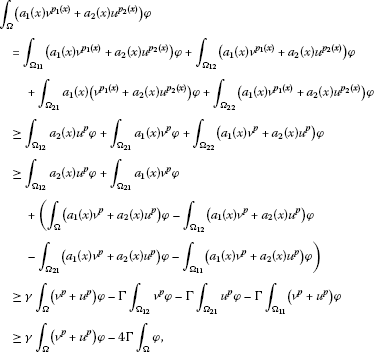where $p=min\left\{{p}_{1}^{-},{p}_{2}^{-}\right\}$ and $\gamma =min\left\{{c}_{1},{c}_{2}\right\}$, $\mathrm{\Gamma }=max\left\{{C}_{1},{C}_{2}\right\}$.

From the convex property of the function $f\left(w\right)={w}^{r}$, $r>1$ and Jensen’s inequality, we obtain

$\begin{array}{rcl}{\int }_{\mathrm{\Omega }}\left({a}_{1}\left(x\right){v}^{{p}_{1}\left(x\right)}+{a}_{2}\left(x\right){u}^{{p}_{2}\left(x\right)}\right)\phi & \ge & \gamma {\int }_{\mathrm{\Omega }}\left({v}^{p}+{u}^{p}\right)\phi -4\mathrm{\Gamma }{\int }_{\mathrm{\Omega }}\phi \\ \ge & \gamma {\int }_{\mathrm{\Omega }}2{\left(\frac{v+u}{2}\right)}^{p}\phi -4\mathrm{\Gamma }{\int }_{\mathrm{\Omega }}\phi \\ \ge & \frac{\gamma }{{2}^{p-1}}{\eta }^{p}\left(t\right)-4\mathrm{\Gamma }.\end{array}$

Then we get

${\eta }^{\prime }\left(t\right)\ge -{\lambda }_{1}\eta \left(t\right)+\frac{\gamma }{{2}^{p-1}}{\eta }^{p}\left(t\right)-4\mathrm{\Gamma }.$

Note that

$\eta \left(t\right)={\int }_{\mathrm{\Omega }}\left(u+v\right)\phi \le \left({\parallel u\left(\cdot ,t\right)\parallel }_{{L}^{\mathrm{\infty }}\left(\mathrm{\Omega }\right)}+{\parallel v\left(\cdot ,t\right)\parallel }_{{L}^{\mathrm{\infty }}\left(\mathrm{\Omega }\right)}\right){\int }_{\mathrm{\Omega }}\phi \le ⦀\left(u,v\right)⦀.$

Hence, for $\eta \left(0\right)$ big enough, the result follows from Lemma 3.1.

Next, we state briefly the proof to the theorem in the case ${f}_{1}\left(u,v\right)={a}_{1}\left(x\right){\int }_{\mathrm{\Omega }}{v}^{{p}_{1}\left(y\right)}\left(y,t\right)\phantom{\rule{0.2em}{0ex}}dy$ and ${f}_{2}\left(u,v\right)={a}_{2}\left(x\right){\int }_{\mathrm{\Omega }}{u}^{{p}_{2}\left(y\right)}\left(y,t\right)\phantom{\rule{0.2em}{0ex}}dy$. We repeat the previous argument under defining $\eta \left(t\right)={\int }_{\mathrm{\Omega }}\left(u+v\right)\phi$, and we obtain in much the same way

${\eta }^{\prime }\left(t\right)\ge -{\lambda }_{1}\eta \left(t\right)+{\int }_{\mathrm{\Omega }}\left({a}_{1}\left(x\right){\int }_{\mathrm{\Omega }}{v}^{{p}_{1}\left(y\right)}\left(y,t\right)\phantom{\rule{0.2em}{0ex}}dy+{a}_{2}\left(x\right){\int }_{\mathrm{\Omega }}{u}^{{p}_{2}\left(y\right)}\left(y,t\right)\phantom{\rule{0.2em}{0ex}}dy\right)\phi \left(x\right)\phantom{\rule{0.2em}{0ex}}dx.$

In view of the property of φ, we get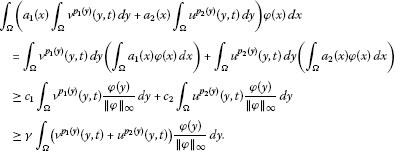According to the convex property of the function $f\left(w\right)={w}^{r}$, $r>1$, and by using Jensen’s inequality, by considering again ${\mathrm{\Omega }}_{11}$, ${\mathrm{\Omega }}_{12}$, ${\mathrm{\Omega }}_{21}$, ${\mathrm{\Omega }}_{22}$ as before, we obtain

$\gamma {\int }_{\mathrm{\Omega }}\left({v}^{{p}_{1}\left(y\right)}\left(y,t\right)+{u}^{{p}_{2}\left(y\right)}\left(y,t\right)\right)\frac{\phi \left(y\right)}{{\parallel \phi \parallel }_{\mathrm{\infty }}}\phantom{\rule{0.2em}{0ex}}dy\ge {\gamma }_{0}{\eta }^{p}\left(t\right)-4\mathrm{\Gamma }|\mathrm{\Omega }|,$

where ${\gamma }_{0}$ depends only on γ, p and ${\parallel \phi \parallel }_{\mathrm{\infty }}$, $|\mathrm{\Omega }|$ denotes the measure of Ω. Hence,

${\eta }^{\prime }\left(t\right)\ge -{\lambda }_{1}\eta \left(t\right)+{\gamma }_{0}{\eta }^{p}\left(t\right)-4\mathrm{\Gamma }|\mathrm{\Omega }|.$

By Lemma 3.1, the proof is complete. □

## 4 Blow-up of solutions for a hyperbolic system

Lemma 4.1 

Let $y\left(t\right)\in {C}^{2}$ satisfying

${y}^{″}\left(t\right)\ge h\left(y\left(t\right)\right),$

$y\left(0\right)=a>0$, ${y}^{\prime }\left(0\right)=b>0$, and $h\left(s\right)\ge 0$ for all $s\ge a$. Then ${y}^{\prime }\left(t\right)>0$ whenever y exists; and

$t\le {\int }_{a}^{y\left(t\right)}{\left({b}^{2}+2{\int }_{a}^{s}h\left(x\right)\phantom{\rule{0.2em}{0ex}}dx\right)}^{-1/2}\phantom{\rule{0.2em}{0ex}}ds.$
(4.1)

Now, let us study the following problem:

$\left\{\begin{array}{c}{u}_{tt}=\mathrm{\Delta }u+{f}_{1}\left(u,v\right),\phantom{\rule{1em}{0ex}}\left(x,t\right)\in {Q}_{T},\hfill \\ {v}_{tt}=\mathrm{\Delta }v+{f}_{2}\left(u,v\right),\phantom{\rule{1em}{0ex}}\left(x,t\right)\in {Q}_{T},\hfill \\ u\left(x,t\right)=0,\phantom{\rule{2em}{0ex}}v\left(x,t\right)=0,\phantom{\rule{1em}{0ex}}\left(x,t\right)\in {S}_{T},\hfill \\ u\left(x,0\right)={u}_{0}\left(x\right),\phantom{\rule{2em}{0ex}}v\left(x,0\right)={v}_{0}\left(x\right),\phantom{\rule{1em}{0ex}}x\in \mathrm{\Omega },\hfill \\ {u}_{t}\left(x,0\right)={u}_{1}\left(x\right),\phantom{\rule{2em}{0ex}}{v}_{t}\left(x,0\right)={v}_{1}\left(x\right),\phantom{\rule{1em}{0ex}}x\in \mathrm{\Omega },\hfill \end{array}$
(4.2)

where ${u}_{0}\left(x\right),{v}_{0}\left(x\right),{u}_{1}\left(x\right),{v}_{1}\left(x\right)\ge 0$ and they are not identically zero, and ${f}_{1}\left(u,v\right)$, ${f}_{2}\left(u,v\right)$ as above respectively.

Theorem 4.1 Let $\left(u,v\right)\in {C}^{2}×{C}^{2}$ be a solution of problem (4.2), and let the conditions in (2.1) hold. Then there exist sufficiently large initial data ${u}_{0}$, ${v}_{0}$, ${u}_{1}$, ${v}_{1}$ such that any solutions of problem (4.1) blew up at finite time ${T}^{\ast }$.

Proof Let $\left({\lambda }_{1},\phi \right)$ be the first eigenvalue and eigenfunction of Laplacian in Ω with homogeneous Dirichlet boundary conditions as before. We assume that ${f}_{1}\left(u,v\right)={a}_{1}\left(x\right){v}^{{p}_{1}\left(x\right)}$, ${f}_{2}\left(u,v\right)={a}_{2}\left(x\right){u}^{{p}_{2}\left(x\right)}$, the other is similar. We also define the function $\eta \left(t\right)={\int }_{\mathrm{\Omega }}\left(u+v\right)\phi$, so we have

$\begin{array}{rcl}{\eta }^{″}\left(t\right)& =& {\int }_{\mathrm{\Omega }}\left({u}_{tt}+{v}_{tt}\right)\phi \\ =& {\int }_{\mathrm{\Omega }}\left(\mathrm{\Delta }u+\mathrm{\Delta }v\right)\phi +{\int }_{\mathrm{\Omega }}\left({a}_{1}\left(x\right){v}^{{p}_{1}\left(x\right)}+{a}_{2}\left(x\right){u}^{{p}_{2}\left(x\right)}\right)\phi \\ =& -{\lambda }_{1}\eta +{\int }_{\mathrm{\Omega }}\left({a}_{1}\left(x\right){v}^{{p}_{1}\left(x\right)}+{a}_{2}\left(x\right){u}^{{p}_{2}\left(x\right)}\right)\phi .\end{array}$

The term ${\int }_{\mathrm{\Omega }}\left({a}_{1}\left(x\right){v}^{{p}_{1}\left(x\right)}+{a}_{2}\left(x\right){u}^{{p}_{2}\left(x\right)}\right)\phi$ is dealt with as before, then we get

${\int }_{\mathrm{\Omega }}\left({a}_{1}\left(x\right){v}^{{p}_{1}\left(x\right)}+{a}_{2}\left(x\right){u}^{{p}_{2}\left(x\right)}\right)\phi \ge \gamma {\int }_{\mathrm{\Omega }}\left({v}^{p}+{u}^{p}\right)\phi -4\mathrm{\Gamma }{\int }_{\mathrm{\Omega }}\phi .$

By virtue of the convex property of the function $f\left(w\right)={w}^{r}$, $r>1$, and Jensen’s inequality, we still obtain

$\gamma {\int }_{\mathrm{\Omega }}\left({v}^{p}+{u}^{p}\right)\phi -4\mathrm{\Gamma }{\int }_{\mathrm{\Omega }}\phi \ge \gamma {\int }_{\mathrm{\Omega }}2{\left(\frac{v+u}{2}\right)}^{p}\phi -4\mathrm{\Gamma }{\int }_{\mathrm{\Omega }}\phi \ge \frac{\gamma }{{2}^{p-1}}{\eta }^{p}\left(t\right)-4\mathrm{\Gamma }.$

Then we have

${\eta }^{″}\left(t\right)\ge -{\lambda }_{1}\eta \left(t\right)+\frac{\gamma }{{2}^{p-1}}{\eta }^{p}\left(t\right)-4\mathrm{\Gamma }.$

Now, we can apply Lemma (4.1) for $a=\eta \left(0\right)$, $b={\eta }^{\prime }\left(0\right)$ large enough such that $-{\lambda }_{1}\eta \left(t\right)+\frac{\gamma }{{2}^{p-1}}{\eta }^{p}\left(t\right)-4\mathrm{\Gamma }>0$, and note that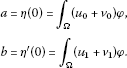Moreover,

$\eta \left(t\right)={\int }_{\mathrm{\Omega }}\left(u+v\right)\phi \le \left({\parallel u\left(\cdot ,t\right)\parallel }_{{L}^{\mathrm{\infty }}\left(\mathrm{\Omega }\right)}+{\parallel v\left(\cdot ,t\right)\parallel }_{{L}^{\mathrm{\infty }}\left(\mathrm{\Omega }\right)}\right){\int }_{\mathrm{\Omega }}\phi \le ⦀\left(u,v\right)⦀.$

Hence, $\left(u,v\right)$ blows up before the maximal time of existence defined in inequality (4.1) is reached. □

## References

1. Chen Y, Levine S, Rao M: Variable exponent, linear growth functions in image restoration. SIAM J. Appl. Math. 2006, 66: 1383-1406. 10.1137/050624522

2. Escobedo M, Herrero MA: Boundedness and blow up for a semilinear reaction-diffusion system. J. Differ. Equ. 1991, 89: 176-202. 10.1016/0022-0396(91)90118-S

3. Escobedo M, Herrero MA: A semilinear parabolic system in a bounded domain. Ann. Mat. Pura Appl. 1998, CLXV: 315-336.

4. Friedman A, Giga Y: A single point blow up for solutions of nonlinear parabolic systems. J. Fac. Sci. Univ. Tokyo Sect. I 1987, 34(1):65-79.

5. Galaktionov VA, Kurdyumov SP, Samarskii AA: A parabolic system of quasiliner equations I. Differ. Equ. 1983, 19(12):2133-2143.

6. Galaktionov VA, Vázquez JL Progress in Nonlinear Differential Equations and Their Applications 56. In A Stability Technique for Evolution Partial Differential Equations. Birkhäuser, Boston; 2004.

7. Kufner A, Oldrich J, Fucik S: Function Space. Kluwer Academic, Dordrecht; 1977.

8. Pinasco JP: Blow-up for parabolic and hyperbolic problems with variable exponents. Nonlinear Anal. 2009, 71: 1094-1099. 10.1016/j.na.2008.11.030

9. Andreu-Vaillo F, Caselles V, Mazón JM Progress in Mathematics 223. In Parabolic Quasilinear Equations Minimizing Linear Growth Functions. Birkhäuser, Basel; 2004.

10. Antontsev, SN, Shmarev, SI: Anisotropic parabolic equations with variable nonlinearity. CMAF, University of Lisbon, Portugal 013, 1-34 (2007)

11. Antontsev, SN, Shmarev, SI: Blow-up of solutions to parabolic equations with nonstandard growth conditions. CMAF, University of Lisbon, Portugal 02, 1-16 (2009)

12. Antontsev SN, Shmarev SI: Parabolic equations with anisotropic nonstandard growth conditions. Int. Ser. Numer. Math. 2007, 154: 33-44. 10.1007/978-3-7643-7719-9_4

13. Antontsev SN, Shmarev S: Blow-up of solutions to parabolic equations with nonstandard growth conditions. J. Comput. Appl. Math. 2010, 234: 2633-2645. 10.1016/j.cam.2010.01.026

14. Erdem D: Blow-up of solutions to quasilinear parabolic equations. Appl. Math. Lett. 1999, 12: 65-69.

15. Glassey RT: Blow-up theorems for nonlinear wave equations. Math. Z. 1973, 132: 183-203. 10.1007/BF01213863

16. Kalashnikov AS: Some problems of the qualitative theory of nonlinear degenerate second-order parabolic equations. Russ. Math. Surv. 1987, 42(2):169-222. 10.1070/RM1987v042n02ABEH001309

17. Levine HA:Some nonexistence and instability theorems for solutions of formally parabolic equations of the form $P{u}_{t}=-Au+F\left(u\right)$. Arch. Ration. Mech. Anal. 1973, 51: 371-386.

18. Levine HA, Payne LE: Nonexistence of global weak solutions for classes of nonlinear wave and parabolic equations. J. Math. Anal. Appl. 1976, 55: 329-334. 10.1016/0022-247X(76)90163-3

19. Lian SZ, Gao WJ, Cao CL, Yuan HJ: Study of the solutions to a model porous medium equation with variable exponents of nonlinearity. J. Math. Anal. Appl. 2008, 342: 27-38. 10.1016/j.jmaa.2007.11.046

20. Ruzicka M Lecture Notes in Math. 1748. In Electrorheological Fluids: Modelling and Mathematical Theory. Springer, Berlin; 2000.

21. Simon J:Compact sets in the space ${L}^{p}\left(0,T;B\right)$. Ann. Mat. Pura Appl. 1987, 4(146):65-96.

22. Tsutsumi M: Existence and nonexistence of global solutions for nonlinear parabolic equations. Publ. Res. Inst. Math. Sci. 1972, 8: 211-229. 10.2977/prims/1195193108

23. Zhao JN:Existence and nonexistence of solutions for ${u}_{t}=div\left({|\mathrm{\nabla }u|}^{p-2}\mathrm{\nabla }u\right)+f\left(\mathrm{\nabla }u,u,x,t\right)$. J. Math. Anal. Appl. 1993, 172: 130-146. 10.1006/jmaa.1993.1012

## Acknowledgements

Supported by NSFC (11271154) and by Key Lab of Symbolic Computation and Knowledge Engineering of Ministry of Education and by the 985 program of Jilin University.

## Author information

Authors

### Corresponding authors

Correspondence to Yunzhu Gao or Wenjie Gao.

### Competing interests

The authors declare that they have no competing interests.

### Authors’ contributions

YG performed the calculations and drafted the manuscript. WG supervised and participated in the design of the study and modified the draft versions. All authors read and approved the final manuscript.

## Rights and permissions

Reprints and Permissions

Gao, Y., Gao, W. Study of solutions to an initial and boundary value problem for certain systems with variable exponents. Bound Value Probl 2013, 76 (2013). https://doi.org/10.1186/1687-2770-2013-76

• Accepted:

• Published:

• DOI: https://doi.org/10.1186/1687-2770-2013-76

### Keywords

• existence
• blow-up
• parabolic system
• hyperbolic system
• variable exponent